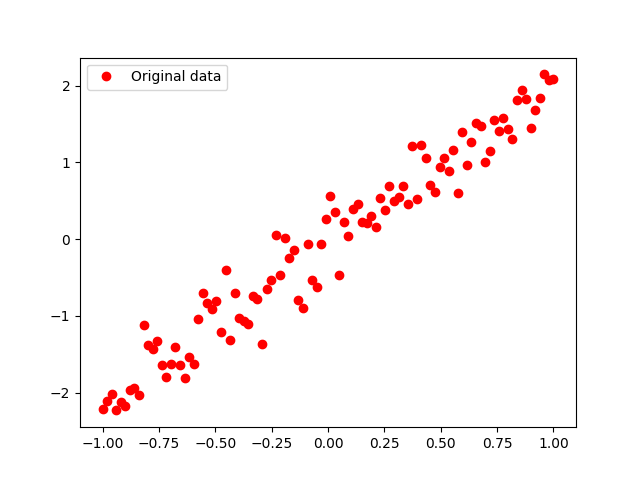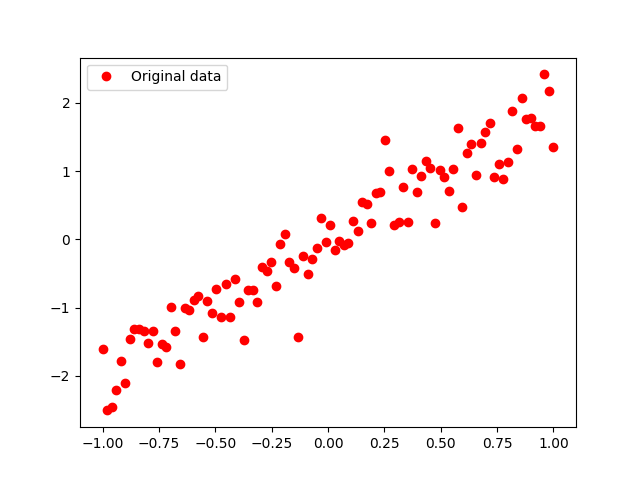# 【tensorflow】制作自己的数据集

🔝🔝🔝🔝🔝🔝🔝🔝🔝🔝🔝🔝🔝🔝🔝🔝🔝

🥰 博客首页：knighthood2001

😗 欢迎点赞👍评论🗨️

❤️ 热爱python，期待与大家一同进步成长！！❤️

# 数据集的基本介绍

数据集是样本的集合，在深度学习中，数据集用于模型训练。再用tensorflow框架开发深度学习模型之前，需要为模型准备好数据集。在训练模型环节，程序需要从数据集中不断地将数据输入模型，模型通过对注入数据的计算来学习特征。

# tensorflow中的数据集

tensorflow中有4种数据集格式

内存对象数据集：直接用字典变量feed_dict，通过注入模式向模型中输入数据。该数据集适用于少量的数据集输入。

TFRecord数据集：用队列式管道（tfrecord）向模型输入数据。该数据集适用大量的数据集输入。

Dataset数据集：通过性能更高的输入管道（tf.data）向模型输入数据。该数据集适用于tensorflow1.4之后的版本。

tf.keras接口数据集：支持tf.keras语法的数据集接口。该数据集适用于tensorflow1.4之后的版本。

# 什么是TFDS

TFDS是tensorflow中的数据集集合模块，该模块将常用的数据及封装起来，实现自动下载与统一的调用接口，为开发模型提供了便利。

## 安装TFDS

``pip install tensorflow-datasets``

## 用TFDS加载数据集

``````import tensorflow_datasets as tfds
print(tfds.list_builders())
ds_train, ds_test = tfds.load(name='mnist', split=["train", "test"])
ds_train = ds_train.shuffle(1000).batch(128).prefetch(10)
for features in ds_train.take(1):
image, label = features["image"], ["label"]``````

``````Downloading and preparing dataset Unknown size (download: Unknown size, generated: Unknown size, total: Unknown size) to ~\tensorflow_datasets\mnist\3.0.1...

Dataset mnist downloaded and prepared to ~\tensorflow_datasets\mnist\3.0.1. Subsequent calls will reuse this data.``````

# 实例：将模拟数据制作成内存对象数据集

本实例将用内存中的模拟数据来制作成数据集，生成的数据集被直接存放在python内存对象中，这样做的好处–数据集的制作可以独立于任何框架

本实例将生成一个模拟y≈2x的数据集，并通过静态图的方式显示出来。

①生成模拟数据

②定义占位符

③建立session会话，获取并显示模拟数据。

④模拟数据可视化

## ①生成模拟数据

在样本制作过程中，最忌讳的是一次性将数据都放入内存中，如果数据量很大，这样容易造成内存用尽，即使是模拟数据，也不建议将数据全部生成以后一次性放入内存中，一般做法是：

Ⅰ创建一个模拟数据生成器，

Ⅱ每次只生成指定批次的样本

下面定义GenerateData函数来生成模拟数据，并将GenerateData函数的返回值设为以生成器方式返回。这种做法使内存被占用的最少

``````import tensorflow as tf
import numpy as np
import matplotlib.pyplot as plt

tf.compat.v1.disable_v2_behavior()

#在内存中生成模拟数据
def GenerateData(batchsize = 100):
train_X = np.linspace(-1, 1, batchsize)   #train_X为-1到1之间连续的100个浮点数
train_Y = 2 * train_X + np.random.randn(*train_X.shape) * 0.3 # y=2x，但是加入了噪声
yield train_X, train_Y       #以生成器的方式返回``````

函数使用yield，使得函数以生成器的方式返回数据。生成器对象只生成一次，过后便会自动销毁，可以省略大量的内存。

## ②定义占位符

``````#定义网络模型结构部分，这里只有占位符张量
Xinput = tf.compat.v1.placeholder("float", (None))
Yinput = tf.compat.v1.placeholder("float", (None))``````

## ③建立session会话，获取并显示模拟数据。

首先定义数据集的迭代次数，接着建立会话，在会话中使用两层for循环；第一层是按照迭代次数来循环，第二层是对GenerateData函数返回的生成器对象进行循环，并将数据打印出来。

因为GenerateData函数返回的生成器对象只有一个元素，所以第二层循环也只运行一次。

``````#建立会话，获取并输出数据
training_epochs = 20  # 定义需要迭代的次数
with tf.compat.v1.Session() as sess:  # 建立会话（session）
for epoch in range(training_epochs): #迭代数据集20遍
for x, y in GenerateData(): #通过for循环打印所有的点
xv,yv = sess.run([Xinput,Yinput],feed_dict={Xinput: x, Yinput: y}) #通过静态图注入的方式，传入数据

print(epoch,"| x.shape:",np.shape(xv),"| x[:3]:",xv[:3])
print(epoch,"| y.shape:",np.shape(yv),"| y[:3]:",yv[:3])``````

## ④模拟数据可视化

``````#显示模拟数据点
train_data = list(GenerateData())
plt.plot(train_data, train_data, 'ro', label='Original data')
plt.legend()
plt.show()``````

## 运行结果

``````...
17 |x.shape: (100,) |x[:3]: [-1.         -0.97979796 -0.959596  ]
17 |y.shape: (100,) |y[:3]: [-2.0945473 -2.1236315 -1.6280223]
18 |x.shape: (100,) |x[:3]: [-1.         -0.97979796 -0.959596  ]
18 |y.shape: (100,) |y[:3]: [-2.022675  -2.118289  -1.8735064]
19 |x.shape: (100,) |x[:3]: [-1.         -0.97979796 -0.959596  ]
19 |y.shape: (100,) |y[:3]: [-2.0080116 -2.5169287 -1.6713679]``````# 改进：创建带有迭代值并支持乱序功能的模拟数据集

①将数据集与 迭代功能绑定在一起，让代码变得更简洁。

②对数据集进行乱序排序，让生成的x数据无规则 。

``pip install sklearn``

``````import tensorflow as tf
import numpy as np
import matplotlib.pyplot as plt
from sklearn.utils import shuffle
tf.compat.v1.disable_v2_behavior()

def GenerateData(training_epochs,batchsize=100):
for i in range(training_epochs):
train_X=np.linspace(-1,1,batchsize)
train_Y=2*train_X+np.random.randn(*train_X.shape)*0.3
yield shuffle(train_X,train_Y),i

Xinput=tf.compat.v1.placeholder("float",(None))
Yinput=tf.compat.v1.placeholder("float",(None))

training_epochs=20
with tf.compat.v1.Session() as sess:
for (x,y),ii in GenerateData(training_epochs):
xv,yv=sess.run([Xinput,Yinput],feed_dict={Xinput:x,Yinput:y})

print(ii,"|x.shape:",np.shape(xv),"|x[:3]:",xv[:3])
print(ii,"|y.shape:",np.shape(yv),"|y[:3]:",yv[:3])

train_data=list(GenerateData(1))
plt.plot(train_data,train_data,'ro',label='Original data')
plt.legend()
plt.show()``````(0)